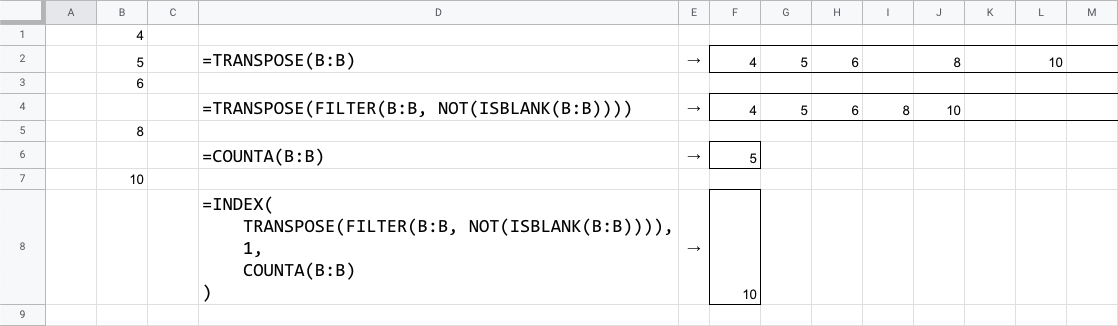# Find the last value in a spreadsheet columnHere is a formula to find the last value in a column of a spreadsheet.

``=INDEX(    TRANSPOSE(FILTER(B:B, NOT(ISBLANK(B:B)))),    1,    COUNTA(B:B))``